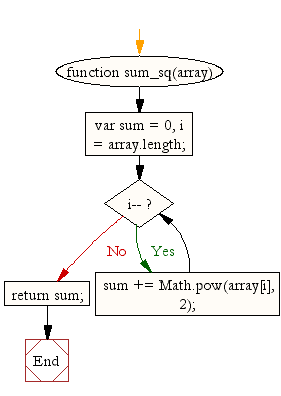# JavaScript: Find the sum of squares of a numeric vector

## JavaScript Array: Exercise-11 with Solution

Write a JavaScript program to find the sum of squares of a numeric vector.

Sample Solution:

HTML Code:

``````<!DOCTYPE html>
<html>
<meta charset=utf-8 />
<title>Sum of squares of a numeric vector</title>
<body>
</body>
</html>
```
```

JavaScript Code:

``````function sum_sq(array) {
var sum = 0,
i = array.length;
while (i--)
sum += Math.pow(array[i], 2);
return sum;
}

console.log(sum_sq([0,1,2,3,4]));
```
```

Sample Output:

```30
```

Flowchart:ES6 Version:

``````function sum_sq(array) {
var sum = 0,
i = array.length;
while (i--) {
sum += Math.pow(array[i], 2);
}return sum;
}

console.log(sum_sq([0, 1, 2, 3, 4]));
``````

Live Demo:

See the Pen JavaScript - Find the sum of squares of a numeric vector- array-ex- 11 by w3resource (@w3resource) on CodePen.

Improve this sample solution and post your code through Disqus

What is the difficulty level of this exercise?

Test your Programming skills with w3resource's quiz.

﻿

## JavaScript: Tips of the Day

Creates an object from the given key-value pairs

Example:

```const tips_objectFromPairs = arr => arr.reduce((a, [key, val]) => ((a[key] = val), a), {});
console.log(tips_objectFromPairs([['x', 2], ['y', 4]]));
```

Output:

```[object Object] {
x: 2,
y: 4
}
```